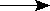Customizing %INDEX ` `

In the course of upgrading the utility routines for Micronetics Standard MUMPS (MSM), version 4.4, the need for an improved version of the utility program ^%INDEX was recognized.

Through the FOIA process, and the kind help of Greg Kreis, a copy of the current version of the VISTA %INDEX software was obtained. (Note from grk: VISTA moved the %INDEX routines into the XIND* namespace. It appears the author of this article has namespaced the routines in %IND* for his use. This is one of those scenarios where a routine name, %INDEX, has been adopted as the product name even after it has been re-namespaced.)

The DVA's (VISTA) software, of course, relies on the services of the Kernel software to support the various utility routines. In order to make ^%INDEX work as a "stand-alone" utility program in MSM, a minor number of modifications was needed to make ^%INDEX work without the support of the Kernel routines.

The text below explains which modifications were made to "customize" the ^%INDEX software for use as a "stand-alone" utility. From the limited list of modifications, it becomes once again clear how important it is to isolate platform-specific code in a small number of subroutines: because the DVA's software follows this premise, the process of "customizing" the software was a simple and very straightforward task.

Ed de Moel
December 1997

### The modifications

1. Calls to ^%ZIS were replaced by new subroutines added to ^%INDEX as follows:
• DO ^%ZISDO %ZIS^%INDEX
• DO ^%ZISCDO %ZISC^%INDEX
• DO HOME^%ZISDO %ZISHOME^%INDEX
2. Calls to NOW^%DTC were replaced by a new subroutine added to ^%INDEX as follows:
• DO NOW^%DTCDO NOW^%INDEX
3. Calls to DD^%DT were replaced by a new subroutine added to ^%INDEX as follows:
• DO DD^%DTDO DDDT^%INDEX
4. References to global variable ^%ZOSF were replaced as follows:
• X ^%ZOSF("UCI")S Y=\$ZU(0)
• ^%ZOSF("VOL")\$SY
(Interesting: there now is an ANSI standard method to obtain a piece of information that used to be non-standard.)
• I \$D(^%ZOSF("RSEL")) X ^("RSEL")D ^%RSEL
• X ^%ZOSF("TEST")I \$D(^ (X))
• X ^%ZOSF("LOAD")S %zosf="S %N=0 X ""ZL @X F XCNP=XCNP+1:1 S %N=%N+1,%=\$T(+%N) Q:\$L(%)=0 S @(DIF_XCNP_"""",0)"""")=%"""
and then later: x %zosf
Interesting: there now also is an ANSI standard method to perform the elementary piece of this action that used to be platform specific:
For I=1:1 Set X=\$Text(+I^@routine) Quit:X="" Set copy(I)=X

With these modifications, the indexer ran on an MSM system without producing any errors.

In order to provide additional (MSM-specific) capabilities, the following modifications were made:

1. Add names of implementation-specific entities to the lists in ^%INDX6
• Z commands
• Z functions
• Z variables
• (There aren't any Z ssvns yet)
2. Make ^%INDX9 recognize names of svns, functions and ssvns when spelled in lower case
3. Add a question to suppress SACC warnings.
• Input option 91 added in ^%INDX6 (in the comments on top of the routine)
• Question added in ^%INDX6
D ANS("Include DVA internal warnings? NO//","NY","Print SACC warnings") G:X="^" END S INP(91)="Yy"[X
• Quit-if code added in A^%INDX1
I 'INP(91),\$E(\$P(%1,";",4),1)="S" Q
4. Make ^%INDX8 recognize names of commands when spelled in lower case
• Added extrinsic function \$\$UCASE
• changed one occurrence of ?1U into ?1A
• Added five references to \$\$UCASE
5. Make ^%INDX8 recognize names of ssvns
6. Make ANS^%INDX6 recognize lower case input for all questions
7. Make ^%INDX2 recognize lower case in names of global and local variables
• At line GLO change ?1U and ?1A
• At line GLO change ?7UN and ?7AN
• At line LOC change ?8UN and ?8AN
• At line LOC change ?7UN and ?7AN
8. Make ^%INDX8 recognize library functions (\$%) and external functions (\$&):
• Test at line FUN becomes:
I " \$\$ \$& \$% "[(" "_\$E(ARG,I,I+1)_" ")

### Further Corrections

In the process of looking at the software, a couple of minor glitches were discovered:

1. In ^%INDX7, the name of ^\$WINDOW was spelled XINDOW
2. In ^%INDX8, the compound goto command just before label GRB is sensitive to changes in the value of local variable X. Changing this line to:
D @\$S("BCHKLMNOPQRUVWZ"[X:"GRB",X="S":"SET","DGX"[X:"DGX","IE"[X:"IFE",X="F":"FOR") S:EOC IDT=IDT-EOC G EE
has the effect that at most one of the possible destinations will be executed, even if the value of local variable X changes in one of these subroutines.

### Initialization

``` k IO
s IOM=80 ; Right margin
s IOSL=50 ; Page length
s IOF="#" ; Form feed _format_
s IO(0)=\$p
```

### %ZIS^%INDEX

```%ZIS d OUT^%SDEV s POP=\$d(QUIT) q:POP
u %DEV s IO=%DEV
s IOM=80 ; Right margin
s IOSL=50 ; Page length
s IOST="" ;
s ION="" ;
s IOF="#" ; Form feed _format_
s IOP="" ;
s IO(0)=\$p
q
```

### %ZISC^%INDEX

```%ZISC C %DEV Q
```

### %ZISHOME^%INDEX

```%ZISHOME U \$p Q
```

### NOW^%INDEX

```NOW S %=\$ZHL(1,"yyyymmdd",\$H)-17000000_"."_\$ZHL(2,"HHmmss",\$P(\$H,",",2)) q
```

### DDDT^%INDEX

```DDDT n d,t
s d=Y\1#100_"-"_\$p("JAN FEB MAR APR MAY JUN JUL AUG SEP OCT NOV DEC"," ",Y\100#100)_"-"_(Y\10000+1700),t=""
S:Y["." t=\$P(Y,".",2)_"0000",t=\$e(t,1,2)_":"_\$e(t,3,4)
S:\$g(%DT)["S" t=\$P(Y,".",2)_"000000",t=\$e(t,1,2)_":"_\$e(t,3,4)_":"_\$e(t,5,6)
S Y=d s:t'="" Y=Y_", "_t
q
```

### UCASE^%INDX8 and UCASE^%INDX9

```UCASE(X) Q \$TR(X,"abcdefghijklmnopqrstuvwxyz","ABCDEFGHIJKLMNOPQRSTUVWXYZ")
```